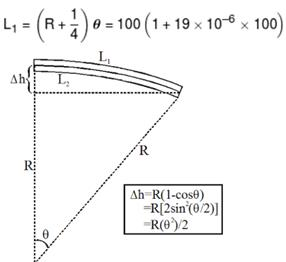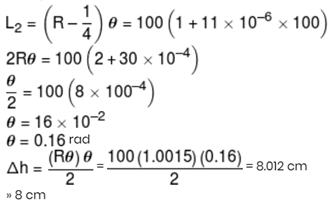Courses

# Physics Test 3 - Units Dimensions And Error Analysis, Kinematics, Laws Of Motion, Rotation, Gravitation, Heat And Thermodynamics

## 25 Questions MCQ Test Mock Test Series for JEE Main & Advanced 2021 | Physics Test 3 - Units Dimensions And Error Analysis, Kinematics, Laws Of Motion, Rotation, Gravitation, Heat And Thermodynamics

Description
This mock test of Physics Test 3 - Units Dimensions And Error Analysis, Kinematics, Laws Of Motion, Rotation, Gravitation, Heat And Thermodynamics for JEE helps you for every JEE entrance exam. This contains 25 Multiple Choice Questions for JEE Physics Test 3 - Units Dimensions And Error Analysis, Kinematics, Laws Of Motion, Rotation, Gravitation, Heat And Thermodynamics (mcq) to study with solutions a complete question bank. The solved questions answers in this Physics Test 3 - Units Dimensions And Error Analysis, Kinematics, Laws Of Motion, Rotation, Gravitation, Heat And Thermodynamics quiz give you a good mix of easy questions and tough questions. JEE students definitely take this Physics Test 3 - Units Dimensions And Error Analysis, Kinematics, Laws Of Motion, Rotation, Gravitation, Heat And Thermodynamics exercise for a better result in the exam. You can find other Physics Test 3 - Units Dimensions And Error Analysis, Kinematics, Laws Of Motion, Rotation, Gravitation, Heat And Thermodynamics extra questions, long questions & short questions for JEE on EduRev as well by searching above.
QUESTION: 1

Solution:
QUESTION: 2

Solution:
QUESTION: 3

### The angle between vectors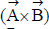and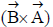is

Solution:
QUESTION: 4

A ball is thrown vertically upward with a speed v from a height h meters above the ground. The time taken for the ball to hit ground is

Solution: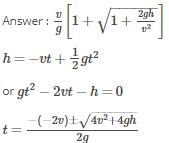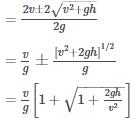Now retain only the positive sign.

QUESTION: 5
A ball rolls from the top of a stair way with a horizontal velocity u m/s. If the steps are h metre high and b metre wide, the ball will hit edge of the nth step, if
Solution: Horizontal dist. travelled=nb Vertical dist. travelled=nh nb=ut nh=0+(1/2)gt^2 => n=(2hu^2)/(gb^2)
QUESTION: 6
Which of the following is the altitude-time graph for a projectile thrown horizontally from the top of the tower?
Solution: When the time is 0 the object will be at a certain height and when we throw the object as the time increases the object will come down ie the altitude decreases.
QUESTION: 7

A long spring is stretched by 2 cm. Its potential energy is U. If the spring is streched by 10 cm, its potential energy would be

Solution:

We know, potential energy of spring is given by (1/2)kx^2 where k is spring constant and x is stretched distance.
Here , x=2cm so, U=(1/2)k*2^2=2k
For, x=10cm :-
E=(1/2)k*10^2=50k=25(2k)=25U.
Final potential energy will be 25U.

QUESTION: 8
A uniform flexible chain of mass m and length 2 l hangs in equilibrium over a smooth horizontal pin of negligible diameter. One end of the chain is given a small vertical displacement so that the chain slips over the pin.The speed of chain when it leaves pin is
Solution:
QUESTION: 9
A stone is tied with a string and is rotated in a circle horizontally. When the string suddenly breaks, the stone will move
Solution:
QUESTION: 10

A sphere A of mass m moving with constant velocity u hits another stationary sphere B of the same mass in a head on collision. if e is the coefficient of restitution, what is the ratio of velocities of sphere A to the sphere B after collision?

Solution:
QUESTION: 11
A boy of mass m is standing on a block of mass M kept on a rough surface. When the boy walks from left to right on the block, the centre of mass (boy block) of system
Solution:
QUESTION: 12

The radius of gyration of a uniform rod of length L about on axis passing through its centre of mass is

Solution:
QUESTION: 13

In case of an orbiting satellite if the radius of orbit is decreased

Solution:

KE=I w²=Iv²/r² ( since omega =v/r)
This implies that KE is inversely proportional to r²
So when radius is decreased , KE will increase due to which PE will decrease
Hence option D is correct.

QUESTION: 14
For a satellite orbiting very close to earth’s surface total energy is
Solution:
QUESTION: 15
A point P (R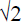,0,0) lies on the axis of a ring of mass M and radius R. The ring is located in y-z plane with its centre at origin O. A small particle of mass m starts from P and reaches O under gravitational attraction only. Its speed of O will be
Solution:
QUESTION: 16
The number of molecules per unit volume of a gas is given by
Solution:
QUESTION: 17

Pressure versus temperature graph of an ideal gas of equal number of moles of different volumes are plotted as shown in figure. Choose the correct alternative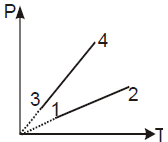Solution:
QUESTION: 18

A system undergoes a cyclic process in which it absorbs Q1 heat and gives out Q2 heat. The efficiency of the process is η and work done is W. Select the correct statement

Solution:

Concept: Carnot engine. Explanation: Heat is transferred to the working material (Q1) and heat is rejected during (Q2). The thermal efficiency is  ηth = W/Q1. Applying first law, we have,  W = Q1 − Q2.  ηth = 1 − Q2/Q1. Hence, correct answer is (b).

QUESTION: 19

A cylinder of radius R, made of a material of thermal conductivity k1,  is surrounded by a cylindrical shell of inner radius R and outer radius 2R. The shell is made of a material of thermal conductivity k2.  The two different temperatures. There is no loss of heat across the cylindrical surface and the system is in steady state. The effective thermal conductivity of the system is

Solution:
QUESTION: 20
If you set up the seventh harmonic on a string fixed at both ends,how many nodes & anti nodes are set up in it
Solution:
*Answer can only contain numeric values
QUESTION: 21

In the arrangement shown coefficient of friction between the two blocks A and B is 0.6 and ground is frictionless. Force constant of spring is 900 N/m. Find the maximum elongation of spring (in cm) such that the blocks do not slip over each other when released.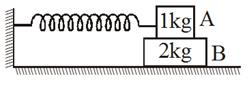Solution:
*Answer can only contain numeric values
QUESTION: 22

Two particles A & B are projected from top of two towers of height 300 m and 600 m with velocity 50 m/s and 250 m/s respectively. The angular velocity of B wrt A is α rad/min. Fill the value of 10a in OMR sheet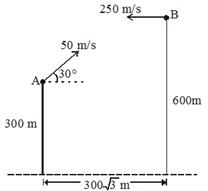Solution: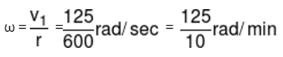*Answer can only contain numeric values
QUESTION: 23

A sphere of radius r is projected up an inclined plane for which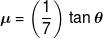with a velocity v0 and initial angular velocity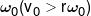. The total time of rise is found to be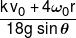Find k.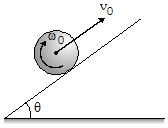Solution:
*Answer can only contain numeric values
QUESTION: 24

A hemispherical bowl of radius R = 0.1m is rotating about its own axis (which is vertical) with an angular velocity ω. A particle of mass 10–2 kg on the frictionless inner surface of the bowl is also rotating with the same ω. The particle is at a height h from the bottom of the bowl. It is desired to measure 'g' using this set up, by measuring h accurately. Assuming that R and ω are known precisely and that the least-count in the measurement of h is 10–4 m, the numerical value of minimum possible error Δg in the measured value of g is given by α x 10–2 m/s2. Fill the value of α in OMR sheet.

Solution: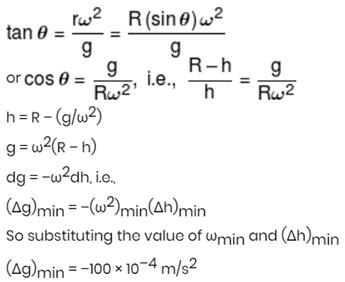*Answer can only contain numeric values
QUESTION: 25

A bimetallic strip consisting of a brass strip and a steel strip, each of length 1 m and each of thickness 0.5 cm is clamped at one end  as shown in figure. Calculate the depression to the nearest integer (in cm) of the free end when it is heated by 100ºC.
[Take : αiron = 11 × 10–6 K–1; αbrass = 19 × 10–6 K–1]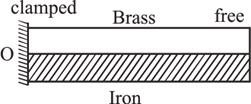Solution: